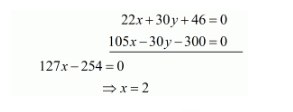# Solve the following systems of equations:`
Question:

Solve the following systems of equations:

$11 x+15 y+23=0$

$7 x-2 y-20=0$

Solution:

The given equations are:

$11 x+15 y+23=0 \quad \ldots(i)$

$7 x-2 y-20=0 \quad \ldots(i i)$

Multiply equation $(i)$ by 2 and equation $(i i)$ by 15 , and add both equations we getPut the value of $x$ in equation $(i)$ we get

$11 \times 2+15 y+23=0$

$\Rightarrow 15 y=-45$

$\Rightarrow y=-3$

Hence the value of $x=2$ and $y=-3$